Related Articles

# Slope of a Straight Line | Class 11 Maths

• Last Updated : 09 Feb, 2021

In mathematics, the slope or gradient of a line is a number that describes both the direction X and Y and the steepness of the line. Slope is denoted by ‘m‘. Slope is calculated by finding the ratio of the “vertical change” to the “horizontal change” between any two distinct points on a line. Sometimes the ratio is expressed as a quotient giving the same number for every two distinct points on the same line.

• A line if it is increasing and it goes up from left to right. The slope is positive, m > 0.
• A line if it is decreasing and it goes down from left to right. The slope is negative, m < 0.
• If the line is horizontal the slope is zero and it is a constant function.
• If a line is vertical, then the slope is undefined.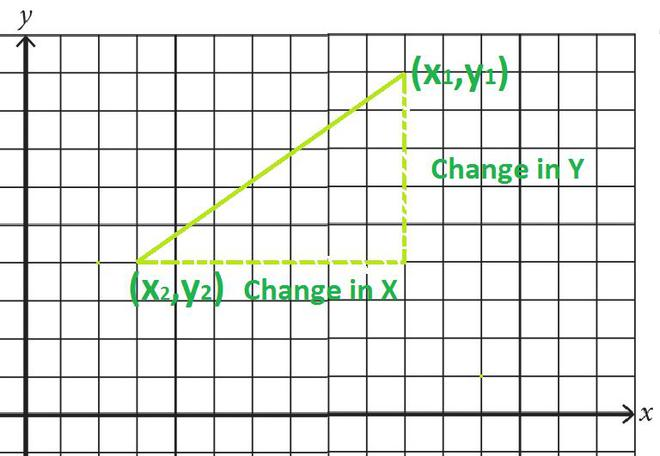In mathematical language, the slope m of the line isThe slope m of the line is related to its angle of incline θ by the tangent function, m = tan(θ)

Thus, a 45-degree rising line has a slope of +1 and a 45-degree falling line has a slope of -1.

### Slope Intercept Form

The linear equation written in the form as

y = mx + c

In the slope-intercept form where:

M is the slope and c is the y-intercept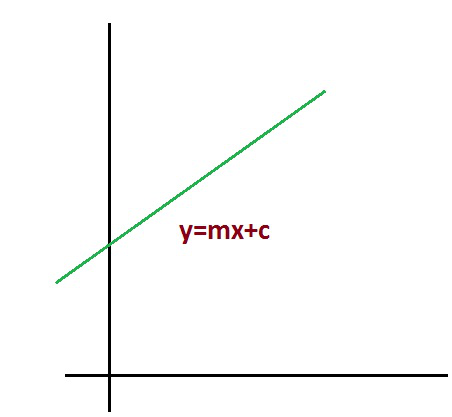Examples of Linear equation in slope-intercept form:

y = 2x + 2

y = 7x – 5

y = 12x + 9

### The Coefficients in Slope-Intercept Form

Slope-intercept form’s advantage is that it gives two main features of the line:

• The slope is m
• The y-coordinate of the y-intercept is b. the y-intercept of the line at (0,b)

For example: a line y = 3x + 1 has slope m as 3 and y – intercept at (0,1)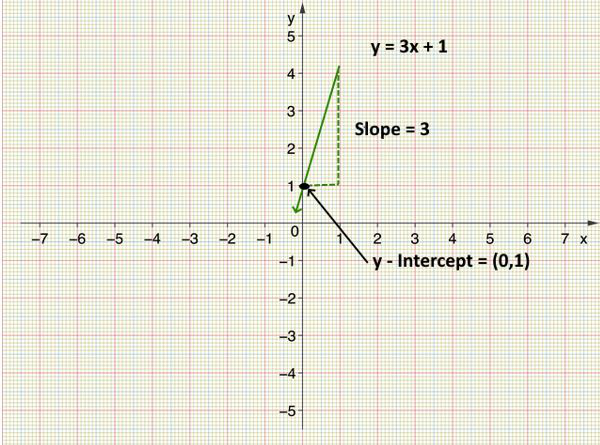This form gives slope (m) and the y-intercept is the reason why it is called as slope-intercept form.

### One Point-Slope Form

If you know the slope m of line and one point (x1, y1) at the line then you can write the equation as y – y1 = m (x – x1) of the line in slope form.

y – y1 = m (x – x1)

This equation is useful, when we know one point on the line and the slope of the line (m) and want to get the other points on the line.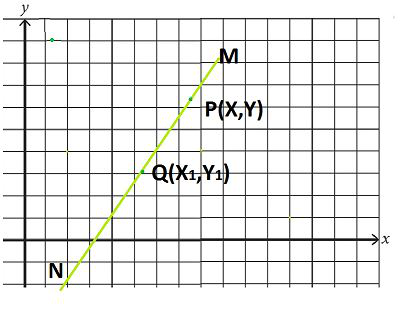This equation is useful, when we know one point on the line and the slope of the line (m) and want to get the other points on line.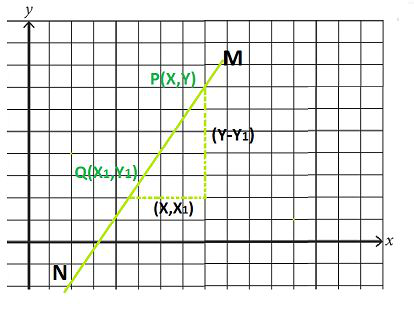Starting with slope,Rearranging like this y – y1 = m (x-x1)

For example: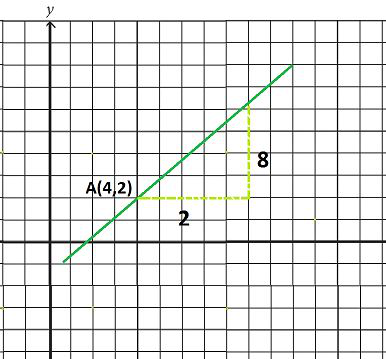Slope, m = 8/2 = 4

y – y1 = m (x – x1)

We know slope m = 4 and point (x1, y1) = (4,2)

Now putting these value in equation

y – 2 = 4 (x – 4)

So;  y – 2 = 4x – 16

y = 4x – 16 +2

y = 4x – 14

### Two Point-Slope Form

Two-point which is given and goes through two-point, says (x1, y1) and (x2, y2). We call this two-point form of the equation of the line.

And two-point form equation given asThis equation used when there is two point on line.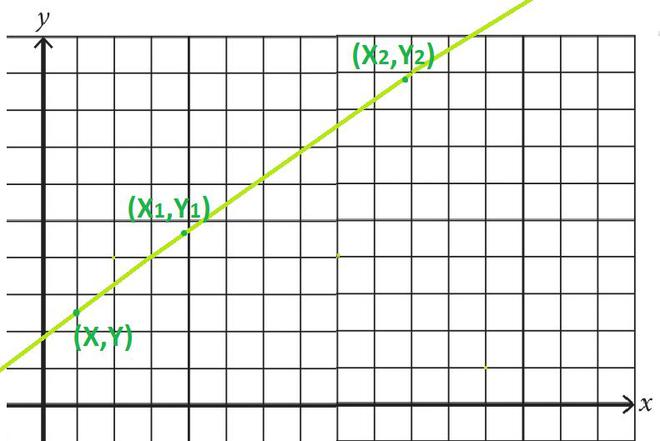Since point A, B and C lies on same line

Slope of AC = slope AB

Now using the two-point slope formula:Multiplying both side by (x – x1)Example: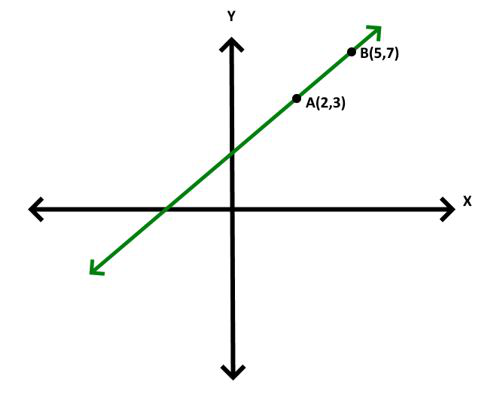Two given points (x1, y1) and (x2, y2) are A (2,3) and B (5,7)3y-9 = 4x-8

3y = 4x+1

### Normal Form of Slope

The equation of the line whose length of the perpendicular from the origin is p and the angle made by the perpendicular with the positive x-axis is given by α is given by:

x cos α + y sin α = p

This is known as the normal form of the line.

In case of the general form of the line Ax + By + C = 0 can be represented in normal form as:From this we can say thatandAlso, it can be inferred that,From the general equation of a straight line Ax + By + C = 0, we can conclude the following:

• The slope is given by -A/B, given that B ≠ 0.

• The x-intercept is given by -C/A and the y-intercept is given by -C/B.

• It can be seen from the above discussion that:• If two points (x1, y1) and (x2, y2) are said to lie on the same side of the line Ax + By + C = 0, then the expressions Ax1+ By1 + C and Ax2 + By2 + C will have the same sign or else these points would lie on the opposite sides of the line.

### Calculating Slope From Graph

For calculating the slope, the formula is given as:Where,

m is the slope of the line.

x1, x2 are the coordinates of x-axis, and

y1, y2 are the coordinates of y-axis.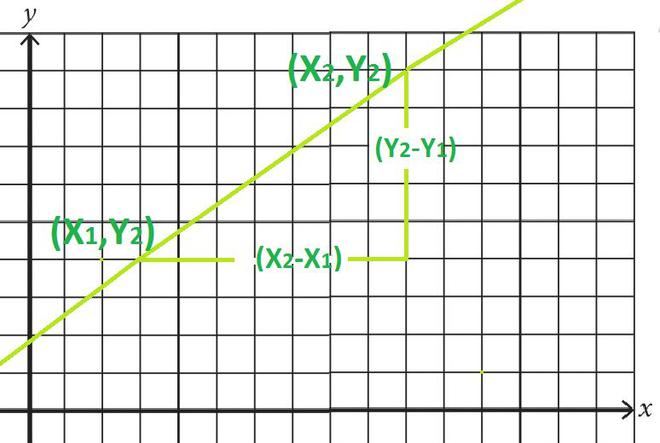Example 1: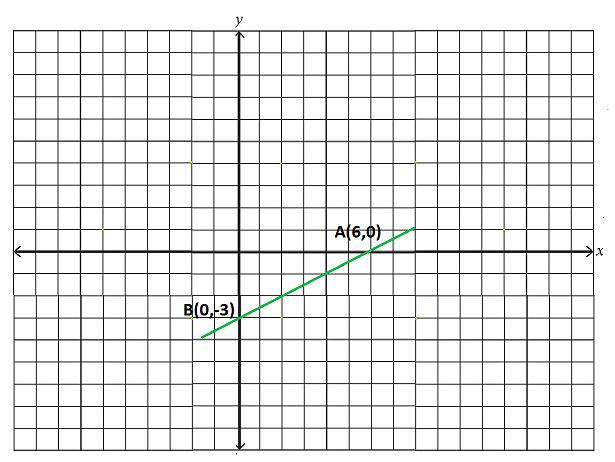Solution:

We have to find Δx and Δy (change in x and change in y)

So change in Δx is 6 and change is Δy  -3

Now slope m isGraphing a Line using given Point and Slope:1. Plot the given point.
2. Use the slope formula to identify the rise and the run.
3. Starting at the given point, count out the rise and run to mark the second point.
4. Connect the points with a line.

Example 2: Graph the line passing through the point (1, -2) and slope m isSolution:

Plot the given point (1, -2)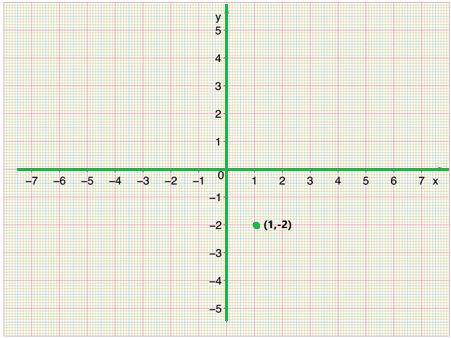Now, use the slope formula to identify the rise and run

We have given slope m isSo, rise is equal to 3 and run is equal to 4

Starting at the point we plotted, count out the rise and run to mark the second point. We count 3 units up and 4 unit’s right.Then we connect the points with a line draw arrow at the ends to show it continues.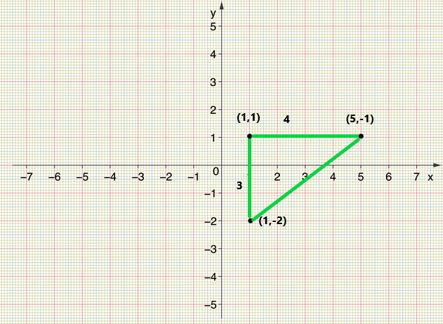We can check our line by starting at any point and counting up 3 and to the right 4. We should get to another point on the line.

### Calculating Slope from Tables

For calculating the slope,

1. Identify the change in each consecutive pair of Y – values in the table.
2. Identify the change in each consecutive pair of X – values in the table.
3. Write ratio showing this corresponding vertical change and the horizontal change.

Example:

Solution:

1. Identify change in each consecutive pair of y so change in the y is 5, 5 and 5.
2. Identify change in each consecutive pair of x so change in the x is 1, 1 and 1.
3. Now writing the ratio using slope formulaand.
4. Simplifying each value we getand. So slope from table is.

Example: Find the point of slope if points are (4, 2) and (8, 12).

Solution:

Given

Point A (4,2) and point B (8,12)

Coordinate x1 and y1 is 4 and 2

Coordinate x2 and y2 is 8 and 12

Now using slope formula for given two pointSLOPE REVIEW:

What is slope?

Slope is measure as the steepness of a line.Change in y and change in x.

### Sample Problems

Question 1. Find the slope of points (1,2) and (2,3).

Solution:

m = (y2 – y1)/(x2 – x1)

= (3 – 2)/(2 – 1)

= 1

Question 2. Find the value of x if slope is 2 and points are (2,2) and (x,6).

Solution:

m = (y2 – y1)/(x2 – x1)

= (6 – 2)/(x – 2)

4 = 2(x-2)

x-2 = 2

x = 4

Question 3. Find the value of y if slope is 3 and points are (2,13) and (4,y).

Solution:

m = (y2 – y1)/(x2 – x1)

= (y – 13)/(4 – 2)

y – 13 = 3(2)

y – 13 = 6

y = 6 + 13 = 19

Question 4. Find the line passing from coordinates (2,5) and slop of line is 5.

Solution:

Slope m = 4

y – y1= m (x – x1)

We know slope m = 5 and point (x1, y1) = (2,5)

Now putting these value in equation

y – 5 = 5 x (x – 2)

So;  y – 5 = 5x – 10

y = 5x – 10 + 5

y = 5x -5

Attention reader! Don’t stop learning now. Join the First-Step-to-DSA Course for Class 9 to 12 students , specifically designed to introduce data structures and algorithms to the class 9 to 12 students

My Personal Notes arrow_drop_up